# Texas Go Math Grade 7 Lesson 6.1 Answer Key Theoretical Probability of Simple Events

Refer to our Texas Go Math Grade 7 Answer Key Pdf to score good marks in the exams. Test yourself by practicing the problems from Texas Go Math Grade 7 Lesson 6.1 Answer Key Theoretical Probability of Simple Events.

## Texas Go Math Grade 7 Lesson 6.1 Answer Key Theoretical Probability of Simple Events

Explore Activity 1

Finding Theoretical Probability

In previous lessons, you found probabilities based on observing data, or experimental probabilities. In this lesson, you will find theoretical probabilities.

At a school fair, you have a choice of spinning Spinner A or Spinner B. You win an MP3 player if the spinner lands on a section with a star in it. Which spinner should you choose if you want a better chance of winning?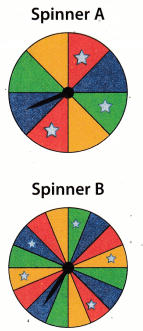A. Complete the table.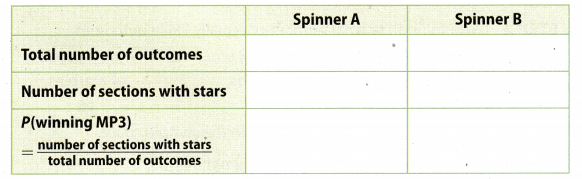B. Compare the ratios for Spinner A and Spinner B.
The ratio for Spinner ________ is greater than the ratio for Spinner _________.
I should choose ________________ for a better chance of winning.

Math Talk
Mathematical Processes

Describe a way to change Spinner B to make your chances of winning equal to your chances of not winning? Explain.

Reflect

Question 1.
Theoretical probability is a way to describe how you found the chance of winning an MP3 player in the scenario above. Using the spinner example to help you, explain in your own words how to find the theoretical probability of an event.
First, find a total number of outcomes for an event
Than find a number of favorable outcomes.
Probability of an event is ratio of the number of favorable outcomes divided by total number of outcomes.

Math Talk
Mathematical Processes

Describe a situation that has a theoretical probability of $$\frac{1}{4}$$.

Question 2.
You roll a number cube one time. What is the probability that you roll a 3 or 4? Write your answer in simplest form.
P(rolling a 3 or 4) =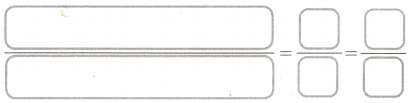P(rolling a 3 or 4) =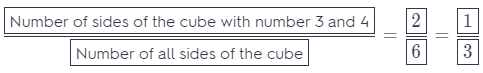The probability that you roll 3 or 4 is $$\frac{1}{3}$$.

Question 3.
How is the sample space for an event related to the formula for theoretical probability?
Size of the sample space is the total number of possible outcomes.

Explore Activity 2

Comparing Theoretical and Experimental Probability

Now that you have calculated theoretical probabilities, you may wonder how theoretical and experimental probabilities compare.Six students are performing in a talent contest. You roll a number cube to determine the order of the performances.
STEP 1: You roll the number cube once. Complete the table of theoretical probabilities for the different outcomes.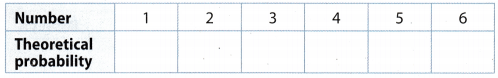STEP 2: Predict the number of times each number will be rolled out of 30 total rolls.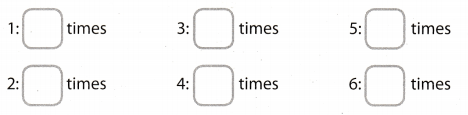STEP 3: Roll a number cube 30 times. Complete the table find its experimental of each number and then find its experimental probability.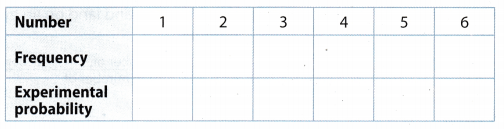STEP 4: Look at the tables you completed. How do the experimental probabilities compare with the theoretical probabilities?

STEP 5: By performing more trials, you tend to get experimental results that are closer to the theoretical probabilities. Combine your frequency results from Step 3 with those of your classmates to make one table for the class. How do the class experimental probabilities compare with the theoretical probabilities?

Reflect

Question 4.
Could the experimental probabilities ever be exactly equal to the theoretical probability? If so, how likely is it? If not, why not?
The theoretical probability is what you expect to happen, but it isn’t always what actually happens. As more trials are conducted, the experimental probability generally gets closer to the theoretical probability.

At a school fair, you have a choice of randomly picking a ball from Basket A or Basket B. Basket A has 5 green balls, 3 red balls, and 8 yellow balls. Basket B has 7 green balls, 4 red balls, and 9 yellow balls. You can win a digital book reader if you pick a red ball. (Explore Activity 1)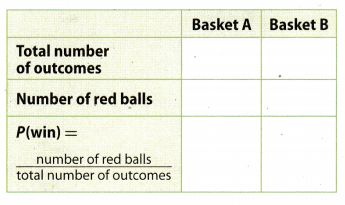Question 1.
Complete the chart. Write each answer in simplest form.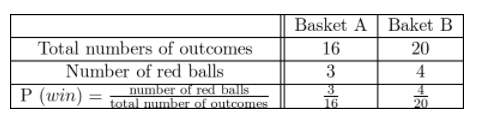Total numbers of outcomes for Basket A is the number of all balls in Basket A – 16.
Total numbers of outcomes for Basket B is the number of all balls in Basket B – 20.
Probability to win ¡s probability to pick red ball from basket. Therefore, probability is number of red balls divided by total number of balls in basket.

Question 2.
Which basket should you choose if you want the better chance of winning?
You should choose Basket B if you want the better chance of winning because probability to pick a red ball, from Basket A is less than probability to pick a red ball from Basket B:
0.18 = $$\frac{3}{16}$$ < $$\frac{4}{20}$$ = 0.20

A spinner has 11 equal-sized sections marked 1 through 11. Find each probability. (Example 1)

Question 3.
You spin once and land on an odd number.P (odd) =P (odd) = $$\frac{6}{11}$$

Question 4.
You spin once and land on an even number.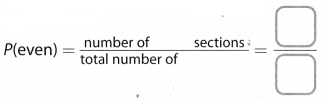P (even) =P (even) = $$\frac{5}{11}$$

You roll a number cube once.

Question 5.
What is the theoretical probability that you roll a 3 or 4? (Example 1)
P (rolling a 3 or 4) =P (rolling a 3 or 4) = $$\frac{1}{3}$$

Question 6.
Suppose you rolled the number cube 199 more times. Would you expect the experimental probability of rolling a 3 or 4 to be the same as your answer to Exercise 5? (Explore Activity 2)
As more trials are conducted, the experimental probability generally gets closer to the theoretical probability. Each experiment has independent throws, which mean if we roll some number that doesn’t conditions the other throws, so it does not have to appear the same answer as in Exercise 5.

Don’ t expect that the experimental probability of rotting a 3 or 4 is the same as the experimental probabiLity in Exercise 5

Essential Question Check-In

Question 7.
How can you find the probability of a simple event if the total number of equally likely outcomes is 20?
If there are 20 equally likely simple events in the sample space, then the probability for each is $$\frac{1}{20}$$.

Find the probability of each event. Write each answer as a fraction in simplest form, as a decimal to the nearest hundredth, and as a percent to the nearest whole number.

Question 8.
You spin the spinner shown. The spinner lands on yellow.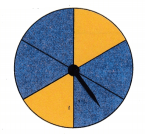P (Spinner land on yellow) =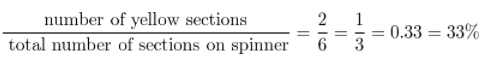Find a decimal and a percent value of fraction.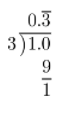The result is: 0.33
Move the decimal point two places to the right and add the ‘ %“ sign.
0.33 = 33%
$$\frac{1}{3}$$ = 33%

Question 9.
You spin the spinner shown. The spinner lands on blue or green.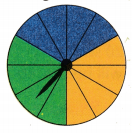P (Spinner land on blue or green) =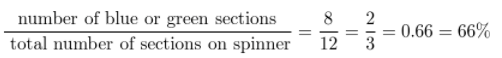Find a decimal and a percent value of fraction.The result is: 0.66
Move the decimal point two places to the right and add the ‘ %“ sign.
0.66 = 66%
$$\frac{2}{3}$$ = 66%

Question 10.
Ajar contains 4 cherry cough drops and 10 honey cough drops. You choose one cough drop without looking. The cough drop is cherry.
P (choose cherry cough drops) =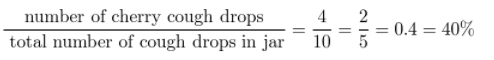Find a decimal and a percent value of fraction.The result is: 0.44
Move the decimal point two places to the right and add the ‘ %“ sign.
0.40 = 40%
$$\frac{2}{5}$$ = 40%

Question 11.
You pick one card at random from a standard deck of 52 playing cards. You pick a black card.
P (picking a black card) =Find a decimal and a percent value of fraction.The result is: 0.50
Move the decimal point two places to the right and add the ‘ %“ sign.
0.50 = 50%
$$\frac{1}{2}$$ = 50%

Question 12.
There are 12 pieces of fruit in a bowl. Five are lemons and the rest are limes. You choose a piece of fruit without looking. The piece of fruit is a lime.
P (choosing a lime) =Find a decimal and a percent value of fraction.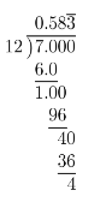The result is: 0.58
Move the decimal point two places to the right and add the ‘ %“ sign.
0.58 = 58%
$$\frac{7}{12}$$ = 58%

Question 13.
You choose a movie CD at random from a case containing 8 comedy CDs, 5 science fiction CDs, and 7 adventure CDs. The CD is not a comedy.The result is: 0.60
Move the decimal point two places to the right and add the ‘ %“ sign.
0.60 = 60%
$$\frac{3}{5}$$ = 60%

Question 14.
You roll a number cube. You roll a number that is greater than 2 and less than 5.
Number greater than 2 and less than 5 is 3 and 4, so we have two numbers that meet this requirement.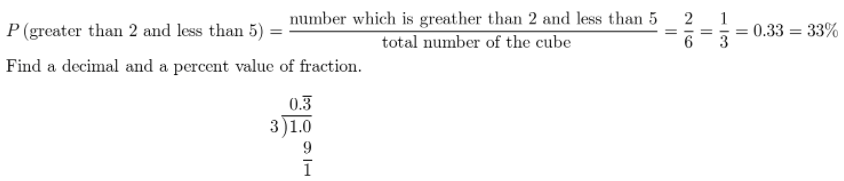The result is: 0.33
Move the decimal point two places to the right and add the ‘ %“ sign.
0.33 = 33%
$$\frac{1}{3}$$ = 33%

Question 15.
Communicate Mathematical ideas The theoretical probability of a given event is $$\frac{9}{13}$$. Explain what each number represents.
Number 9 represent number of ways the event can occur and 13 represent total number if equally likely outcomes.

Question 16.
Leona has 4 nickels, 6 pennies, 4 dimes, and 2 quarters in a change purse. Leona lets her little sister Daisy pick a coin at random. If Daisy is equally likely to pick each type of coin, what is the probability that her coin is worth more than five cents? Explain.
Penny worth 1 cent, Nickel. 5 cents, Dime 10 cents and quarter worth 25 cents. First, find a total number of coins that worth more than 5 cents. Those are nickles, dimes and quarters.
Than find a number of all coins. It is 16. Probability to pick more than five cents is:
P (more than five cents) =P (more than five cents) = $$\frac{5}{8}$$

H.O.T. Focus on Higher Order Thinking

Question 17.
Critique Reasoning A bowl of flower seeds contains 5 petunia seeds and 15 begonia seeds. Riley calculated the probability that a randomly selected seed is a petunia seed as $$\frac{1}{3}$$. Describe and correct Riley’s error.
Riley’s error: He divided number of petunia seeds by number of begonia seeds.
P (selecting seed of petunia) =P (selecting seed of petunia) = $$\frac{1}{4}$$

Question 18.
There are 20 seventh graders and 15 eighth graders in a club. A club president will be chosen at random.
a. Analyze Relationships Compare the probabilities of choosing a seventh grader or an eighth grader.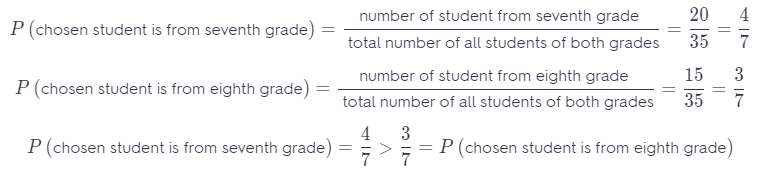b. Critical Thinking If a student from one grade is more likely to be chosen than a student from the other, is the method unfair? Explain.
There are more students from seventh grade than students from eighth grade, but how we chose at random this method is not unfair.

A jar contains 8 red marbles, 10 blue ones, and 2 yellow ones. One marble is chosen at random. The color is recorded in the table, and then it is returned to the jar. This is repeated 40 times.Question 19.
Communicate Mathematical Ideas Use proportional reasoning to explain how you know that for each color, the theoretical and experimental probabilities are not the same.
The theoretical probability is: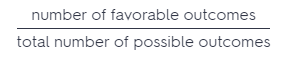The experimental probability is: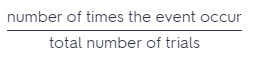In this exercise total number of possible outcomes and totaL number of trials is the same because we have 40 marbles and 40 trials.

Number of favorable outcomes are determined with number of each color, while number of times the event occur can be different from one experiment to other experiment.

In order for probabilities to be the same in this exercise, the number of favorable outcomes should be equal to the number of times the event occur, but it does not matter in the general case.

Question 20.
Persevere in Problem Solving For which color marble is the experimental probability closest to the theoretical probability? Explain.